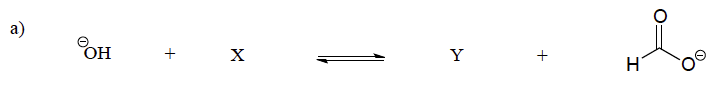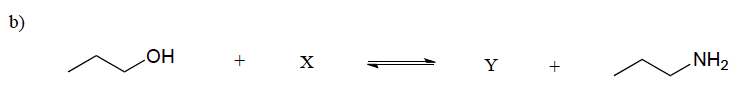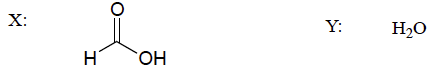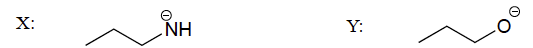# 3.1: Acids and Bases - The Brønsted-Lowry Definition

$$\newcommand{\vecs}{\overset { \rightharpoonup} {\mathbf{#1}} }$$ $$\newcommand{\vecd}{\overset{-\!-\!\rightharpoonup}{\vphantom{a}\smash {#1}}}$$$$\newcommand{\id}{\mathrm{id}}$$ $$\newcommand{\Span}{\mathrm{span}}$$ $$\newcommand{\kernel}{\mathrm{null}\,}$$ $$\newcommand{\range}{\mathrm{range}\,}$$ $$\newcommand{\RealPart}{\mathrm{Re}}$$ $$\newcommand{\ImaginaryPart}{\mathrm{Im}}$$ $$\newcommand{\Argument}{\mathrm{Arg}}$$ $$\newcommand{\norm}{\| #1 \|}$$ $$\newcommand{\inner}{\langle #1, #2 \rangle}$$ $$\newcommand{\Span}{\mathrm{span}}$$ $$\newcommand{\id}{\mathrm{id}}$$ $$\newcommand{\Span}{\mathrm{span}}$$ $$\newcommand{\kernel}{\mathrm{null}\,}$$ $$\newcommand{\range}{\mathrm{range}\,}$$ $$\newcommand{\RealPart}{\mathrm{Re}}$$ $$\newcommand{\ImaginaryPart}{\mathrm{Im}}$$ $$\newcommand{\Argument}{\mathrm{Arg}}$$ $$\newcommand{\norm}{\| #1 \|}$$ $$\newcommand{\inner}{\langle #1, #2 \rangle}$$ $$\newcommand{\Span}{\mathrm{span}}$$

##### Objectives

After completing this section, you should be able to

1. state the Brønsted-Lowry definition of an acid and a base.
2. identify the Brønsted-Lowry acid and base in a given acid-base reaction.
3. identify the conjugate base of an acid and identify the conjugate acid of a base.
##### Key Terms

Make certain that you can define, and use in context, the key terms below.

• Brønsted-Lowry acid
• Brønsted-Lowry base
• conjugate acid
• conjugate base
##### Study Notes

You should already be familiar with the Brønsted-Lowry concept of acidity and the differences between strong and weak acids. You may wish to review this topic before proceeding.

In 1923, chemists Johannes Brønsted and Martin Lowry independently developed definitions of acids and bases based on compounds abilities to either donate or accept protons (H+ ions). Here, acids are defined as being able to donate protons in the form of hydrogen ions; whereas bases are defined as being able to accept protons. This took the Arrhenius definition one step further as water is no longer required to be present in the solution for acid and base reactions to occur.

## Brønsted-Lowry DefinitionJ.N. Brønsted and T.M. Lowry independently developed the theory of proton donors and proton acceptors in acid-base reactions, coincidentally in the same region and during the same year. The Arrhenius theory where acids and bases are defined by whether the molecule produces hydrogen ion or hydroxide ion when dissolved in water was too limiting, because not all chemical reactions, especially organic reactions, occur in water. The Brønsted-Lowry Theory defines an acid a proton donor, while a base is a proton acceptor. This is illustrated in the following reactions:

$HCl + HOH \rightarrow H_3O^+ + Cl^-$

$HOH + NH_3 \rightarrow NH_4^+ + OH^-$

Acid Base
Donates hydrogen ions Accepts hydrogen ions.
HCl HOH → H3O+ + Cl
HOH NH3 NH4+ + OH

The determination of a substance as a Brønsted-Lowry acid or base can only be done by examining the reaction, since many chemicals can be either an acid or a base. For example, HOH is a base in the first reaction and an acid in the second reaction.

Bronsted-Lowry Acids and BasesTo determine whether a substance is an acid or a base, count the hydrogens on each substance before and after the reaction. If the number of hydrogens has decreased, then that substance is the acid (donates hydrogen ions). If the number of hydrogens has increased, then that substance is the base (accepts hydrogen ions). These definitions are normally applied to the reactants on the left. If the reaction is viewed in reverse a new acid and base can be identified. The substances on the right side of the equation are called the conjugate acid and conjugate base compared to those on the left. Also note that an acid turns into a conjugate base, and the base turns into the conjugate acid after the reaction is over.

## Conjugate Acid–Base PairsIn aqueous solutions, acids and bases can be defined in terms of the transfer of a proton from an acid to a base. Thus for every acidic species in an aqueous solution, there exists a species derived from the acid by the loss of a proton. These two species that differ by only a proton constitute a conjugate acid–base pair. For example, in the reaction of HCl with water shown below, HCl , the parent acid, donates a proton to a water molecule, the parent base, thereby forming Cl-. Thus HCl and Cl- constitute a conjugate acid–base pair. By convention, we always write a conjugate acid–base pair as the acid followed by its conjugate base. In the reverse reaction, the Cl- ion in solution acts as a base to accept a proton from H3O+, forming H2O and HCl. Thus H3O+ and H2O constitute a second conjugate acid–base pair. In general, any acid–base reaction must contain two conjugate acid–base pairs, which in this case are HCl/Cl- and H3O+/H2O.All acid–base reactions contain two conjugate acid–base pairs.

Similarly, in the reaction of acetic acid with water, acetic acid donates a proton to water, which acts as the base. In the reverse reaction, H3O+ is the acid that donates a proton to the acetate ion, which acts as the base. Once again, we have two conjugate acid–base pairs: the parent acid and its conjugate base (CH3CO2H/CH3CO2-) and the parent base and its conjugate acid (H3O+/H2O).In the reaction of ammonia with water to give ammonium ions and hydroxide ions, ammonia acts as a base by accepting a proton from a water molecule, which in this case means that water is acting as an acid. In the reverse reaction, an ammonium ion acts as an acid by donating a proton to a hydroxide ion, and the hydroxide ion acts as a base. The conjugate acid–base pairs for this reaction are NH4+/NH3 and H2O/OH-.##### Example $$\PageIndex{1}$$

Aniline (C6H5NH2) is slightly soluble in water. It has a nitrogen atom that can accept a hydrogen ion from a water molecule just like the nitrogen atom in ammonia does. Write the chemical equation for this reaction and identify the Brønsted-Lowry acid and base.

Solution

C6H5NH2 and H2O are the reactants. When C6H5NH2 accepts a proton from H2O, it gains an extra H and a positive charge and leaves an OH ion behind. The reaction is as follows:

$\ce{C6H5NH2(aq) + H2O(ℓ) <=> C6H5NH3^{+}(aq) + OH^{−}(aq)} \nonumber$

Because C6H5NH2 accepts a proton, it is the Brønsted-Lowry base. The H2O molecule, because it donates a proton, is the Brønsted-Lowry acid.

##### Example $$\PageIndex{1}$$

Identify the conjugate acid-base pairs in this equilibrium.

$(CH_{3})_{3}N + H_{2}O\rightleftharpoons (CH_{3})_{3}NH^{+} + OH^{-} \nonumber$

Solution

One pair is H2O and OH, where H2O has one more H+ and is the conjugate acid, while OH has one less H+ and is the conjugate base.

The other pair consists of (CH3)3N and (CH3)3NH+, where (CH3)3NH+ is the conjugate acid (it has an additional proton) and (CH3)3N is the conjugate base.

Some common conjugate acid–base pairs are shown in Figure 2.7.1. The strongest acids are at the bottom left, and the strongest bases are at the top right. The conjugate base of a strong acid is a very weak base, and, conversely, the conjugate acid of a strong base is a very weak acid.

Figure 2.7.1 The Relative Strengths of Some Common Conjugate Acid–Base Pairs## Exercises

1. Identify the Brønsted-Lowry acids and bases in the reactions given below.
1. $$CH3CH2O^- + H2O <=> CH3CH2OH + OH^-$$
2. $$CH3CH2OH + H2SO4 <=> CH3CH2OH2+ + HSO4-$$
2. Show the structures of species X and Y in the following acid-base reactions:1.2.1. a)b)#### Questions

Q2.7.1

Is the following molecule a Brønsted acid or base?

HSO4

#### Solutions

S2.7.1

It can be both, consider the following schemes: